## RS Aggarwal Class 8 Solutions Chapter 1 Rational Numbers Ex 1H

These Solutions are part of RS Aggarwal Solutions Class 8. Here we have given RS Aggarwal Solutions Class 8 Chapter 1 Rational Numbers Ex 1H.

Other Exercises

Objective Questions :
Tick the correct answer in each of the following :

Question 1.
Solution: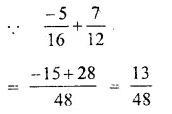Question 2.
Solution: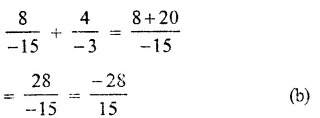Question 3.
Solution: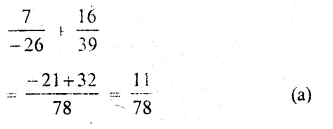Question 4.
Solution:Question 5.
Solution: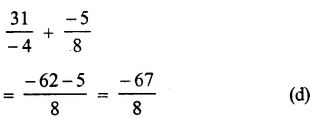Question 6.
Solution:Question 7.
Solution: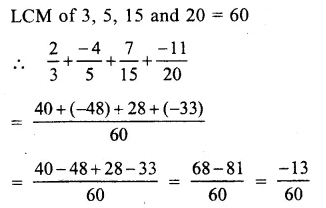Question 8.
Solution: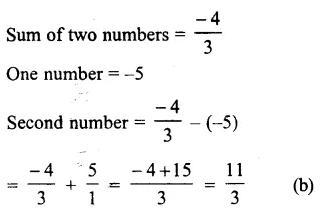Question 9.
Solution: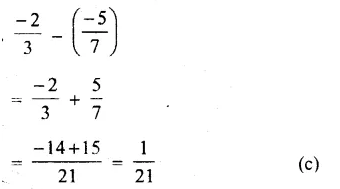Question 10.
Solution: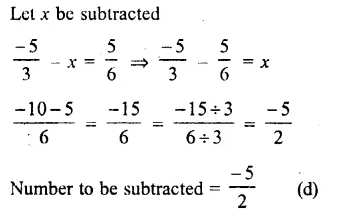Question 11.
Solution: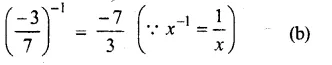Question 12.
Solution:
Product of two numbers = $$\\ \frac { -28 }{ 81 }$$
One number = $$\\ \frac { 14 }{ 27 }$$Question 13.

Solution:
Let x be the required number, then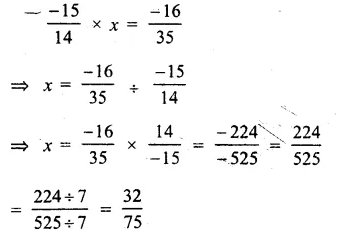Question 14.
Solution:
Let x is to be subtracted then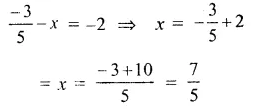Question 15.
Solution:
sum = -3,one number = $$\\ \frac { -10 }{ 3 }$$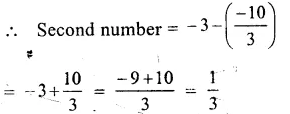Question 16.
Solution:
We know that a number is called in standard form if the numerator and denominator has no common divisor except 1.
$$\\ \frac { -9 }{ 6 }$$ is in standard form.

Question 17.
Solution:Question 18.
Solution:Question 19.
Solution:
Let x is required rationalQuestion 20.
Solution:
Additive inverse of $$\\ \frac { -5 }{ 9 }$$ is = – $$\left( \frac { -5 }{ 9 } \right)$$

Question 21.
Solution:
Reciprocal of $$\\ \frac { -3 }{ 4 }$$ is $$\\ \frac { -4 }{ 3 }$$

Question 22.
Solution:
A rational number between = $$\\ \frac { -2 }{ 3 }$$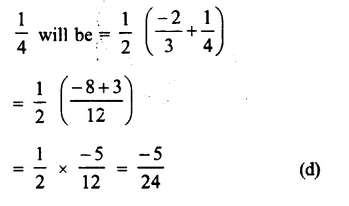Question 23.

Solution: